# Discrete Mathematics and Algorithms Research

The Department has a strong faculty working in various topics in discrete mathematics, especially algorithmic aspects. The interface between Theoretical Computer Science and Discrete Mathematics has been highlighted by recent EPSRC Reviews of both disciplines as being one of increasing importance. LSE is well placed to be at the forefront of future developments.

Individual faculty interests are listed below, along with our Postdocs and Research students.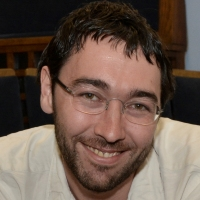Extremal and probabilistic combinatorics; Ramsey theory; graph and hypergraph theory; quasirandom structures; algorithmic combinatorics

Professor Martin Anthony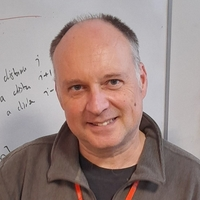Mathematical aspects of machine learning; probabilistic modelling of learning; discrete mathematical problems in the theory of learning; Boolean function classes and their representationsAlgorithms and the theory of computation; randomised algorithms; sublinear algorithms on massive data sets; property testing; computational statistics

Professor Julia Böttcher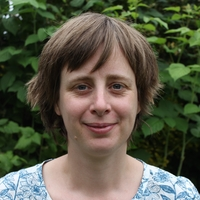Extremal combinatorics; random discrete structures; Ramsey theory; regularity method; graph packing; quasirandomness; algorithmic aspects in combinatorics

Professor Graham BrightwellCombinatorics; finite partially ordered sets; probabilistic methods; algorithmic aspects in combinatorics; random graphs; random structures

Professor Jan van den HeuvelGraph theory; matroid theory; applications and algorithmic aspects of graph theory; mathematical aspects of frequency assignment problems; network science

Professor Andrew Lewis-PyeLogic; computability; discrete mathematics; algorithmic randomness; network science; complex systems; distributed computing; cryptocurrencies

Dr Emilio Pierro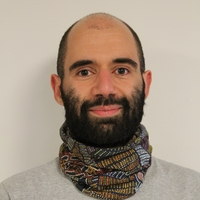Finite group theory; groups of Lie type; geometric group theory; combinatorics; generation of finite simple groups

Dr Robert SimonMeasure theory; ergodic theory; functional analysis; stochastic processes; chaos theory; combinatorics; algebraic topology

Professor Jozef Skokan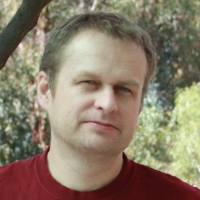Extremal set theory; quasirandom structures; probabilistic combinatorics; discrete geometry; graph theory; combinatorial games; topics in theoretical computer science